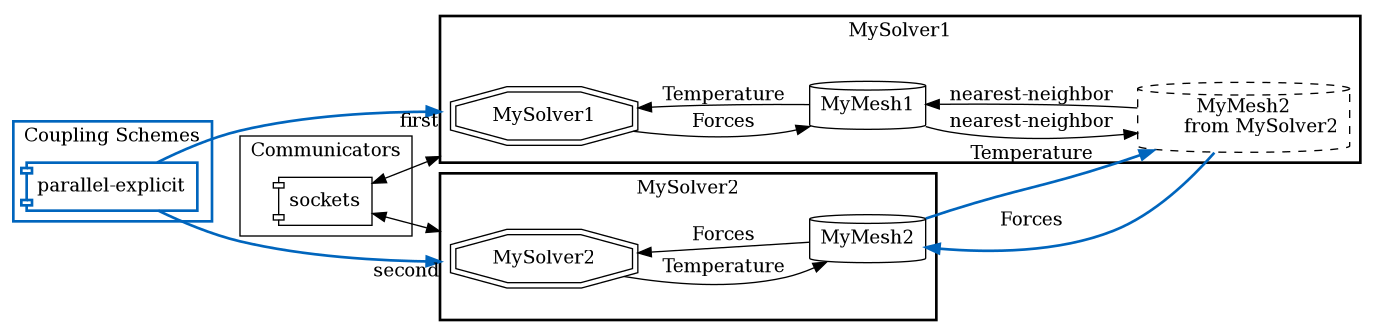The coupling scheme is the centerpiece of the preCICE configuration. It describes the logical execution order of two or more participants. On this page, we explain how to couple two participants.

A coupling scheme can be either serial or parallel and either explicit or implicit. Serial refers to the staggered execution of one participant after the other. Parallel, on the other hand, refers to the simultaneous execution of both participants. With an explicit scheme, both participants are only executed once per time window. With an implicit scheme, the participants are executed multiple times until convergence.

For coupling more than two participants, please see the page on multi coupling.## Explicit coupling schemes

For a serial-explicit coupling scheme, your configuration could look like this:

<coupling-scheme:serial-explicit>
<participants first="MySolver1" second="MySolver2"/>
<max-time-windows value="20"/>
<time-window-size value="1e-3"/>
<exchange data="Forces" mesh="MyMesh2" from="MySolver1" to="MySolver2"/>
<exchange data="Temperature" mesh="MyMesh2" from="MySolver2" to="MySolver1"/>
</coupling-scheme:serial-explicit>


With the participants tag, you define which participants are coupled in this scheme. For a serial scheme, the first participant is computed before the second one. For a parallel-explicit scheme, write:

<coupling-scheme:parallel-explicit>
<participants first="MySolver1" second="MySolver2"/>
...
</coupling-scheme:parallel-explicit>


Now, the first and the second participant are executed at the same time. Actually, it makes no real differences who is who here (it will make a difference for implicit coupling, but that is described further down).

With max-time-windows, you say how many coupling time windows you want to simulate. Alternatively, you can use:

<max-time value="1.0"/>


Afterwards,

precice.isCouplingOngoing()


will return false and precice.finalize() should be called (compare with step 5 of the couple-your-code section).

With time-window-size, you can define the coupling time window (=coupling time step) size. If a participant uses a smaller one, it will subcycle until this window size is reached. Find more details also in step 5 of the couple-your-code section.

Finally, with exchange, you need to define which data values should be exchanged within this coupling scheme:

<exchange data="Forces" mesh="MyMesh2" from="MySolver1" to="MySolver2"/>


mesh needs to be a mesh that both participant use, typically one participant provides the mesh and the other receives it, as we explained on the introduction page. If this still confuses you have a look at the mesh exchange example.

## Implicit coupling schemes

For implicit coupling, you need to specify several additional options:

<coupling-scheme:parallel-implicit>
<participants first="MySolver1" second="MySolver2"/>
...
<exchange data="Temperature" mesh="MyMesh2" from="MySolver2" to="MySolver1"/>
<max-iterations value="100"/>
<relative-convergence-measure limit="1e-4" data="Displacements" mesh="MyMesh2"/>
<relative-convergence-measure limit="1e-4" data="Forces" mesh="MyMesh2"/>
<acceleration:IQN-ILS>
...
</acceleration:IQN-ILS>
</coupling-scheme:parallel-implicit>


To control the number of sub-iterations within an implicit coupling loop, you can specify the maximum number of iterations, max-iterations and you can specify one or several convergence measures:

• relative-convergence-measure for a relative criterion
• absolute-convergence-measure for an absolute criterion
• min-iteration-convergence-measure to require a minimum of iterations
If multiple convergence measure are combined they all need to be fulfilled to go to the next time window. Alternatively, you can specify suffices="yes" within any convergence measure. The data used for a convergence measure needs to be exchanged within the coupling-scheme (tag exchange).

Each convergence measure prints its current state as INFO logging in every coupling iteration (how to configure the logging). For example for a relative-convergence-measure:

relative convergence measure: relative two-norm diff = 2.6023e-05, limit = 1e-05, normalization = 0.00100051, conv = false

• relative two-norm diff is the relative coupling residual ||H(x^k) - x^k||_2 / ||x^k||_2 for a fixed-point equation H.
• limit is the convergence limit specified in the configuration.
• normalization is the normalization factor ||x^k||_2.

Most important for implicit coupling is to use a acceleration scheme, i.e. to let preCICE modify the exchanged data. We give more details on the acceleration configuration page. For numerical reasons, you should always use a acceleration for implicit coupling. Otherwise, an implicit coupling has no benefit over an explicit coupling. You can only define one acceleration per coupling scheme.

Additionally, you can speed up an implicit coupling by using an extrapolated value from previous time windows as initial guess, <extrapolation-order value="2"/>. This tag is optional and requires some trial-and-error tuning as extrapolation does not always result in fewer iterations. Use with care!

For implicit coupling, the tags first and second do not only determine the order of execution (for serial coupling), but they also determine where preCICE computes the convergence measures and the acceleration: Both are executed on the second participant.

Besides parallel-implicit, you can also use a serial-implicit coupling. However, for performance reasons, we recommend to use parallel-implicit. To explain this is beyond the scope of this documentation. We refer, instead, to the respective publications.

Did you know, you can also inspect the number of iterations and the residuals through log files? Have a look at the output files description.